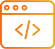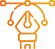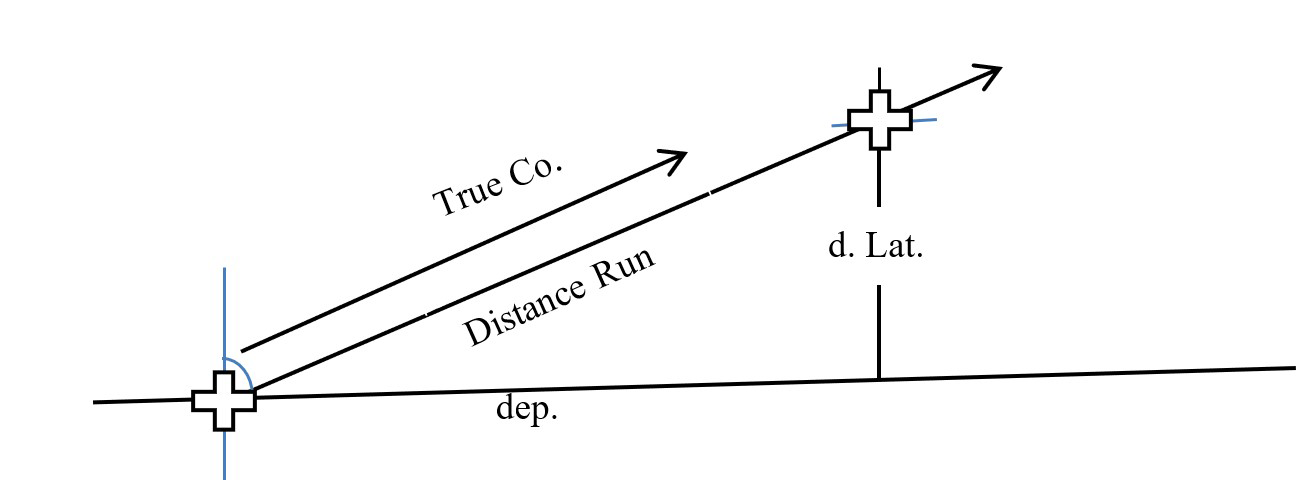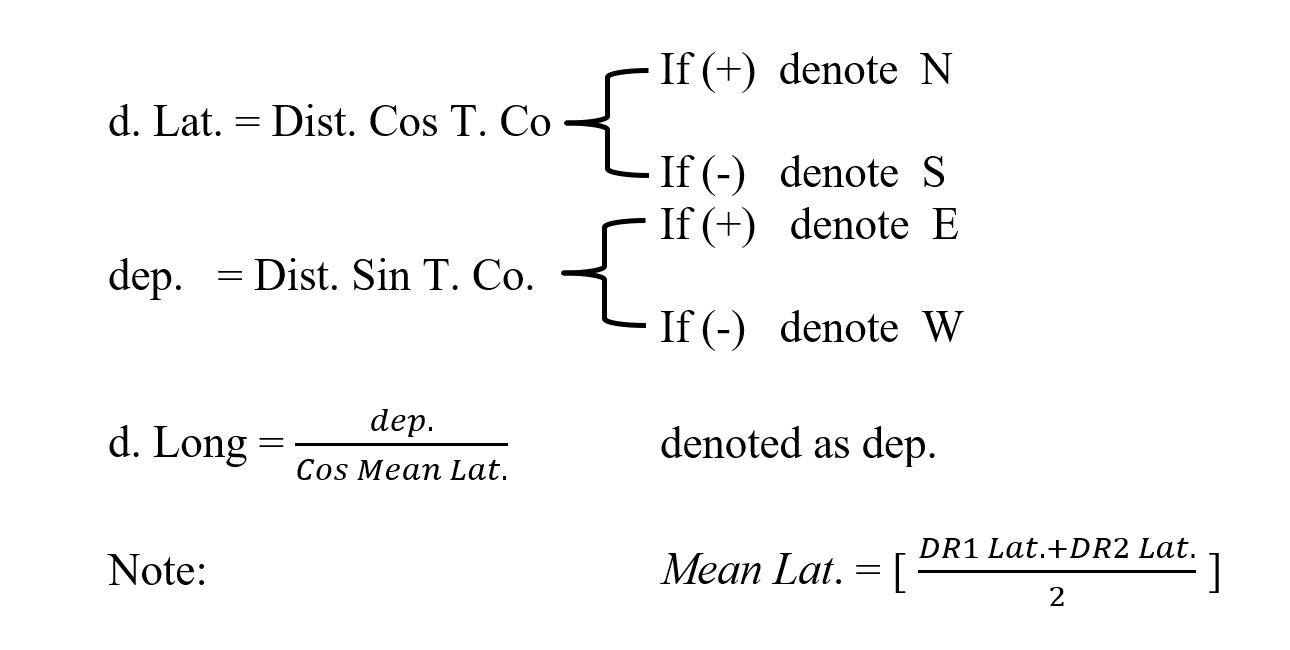### Finding the ship's arrival position

While solving the exercises, it may be necessary to find the final position of the ship, given the following determinants.

• Initial position
• True course
• Distance run

Therefore, this program is designed to solve this issue by applying well-known sailing laws, which we summarize as follows

Apply it now### Finding the True Course and the Distance Run from Position (A) to Position (B):

While solving the exercises, it may be necessary to find the course and distance between two positions of the ship, given the following determinants.

• Position (A).
• Position (B).

Therefore, this program is designed to solve this issue by applying the Mercator rules, which we summarize as follows

• Solving for difference in Latitude (d Lat.)
• Solving for difference in Longitude (d Long.)
• Solving for Meridional Parts and difference in Meridional Parts (DMP)
• Tan Course = (d Long.) / (DMP)
• Distance = (d Lat.) / Cos Course
Apply it now